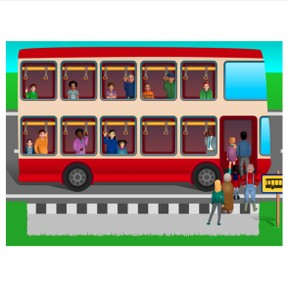On the bus: Addition to 20

# On the bus: Addition to 208,000 schools use Gynzy92,000 teachers use Gynzy1,600,000 students use Gynzy

## General

Students learn to add to 20 using the context of a bus ride without carrying numbers.

## Relevance

If students want to be able to calculate quickly and easily, it is important to learn to add to 20. The bus context helps students understand how addition works and can support that learning.

## Introduction

Show the image of the double decker bus and ask if anyone in class has ever been on a double decker bus. Show the next page. It shows a double decker bus next to two regular busses stacked on top of each other. Explain that this type of bus is called a double decker and see if they can figure out why. Then ask students to count amounts by asking students to count the number of children waiting for the bus in the images.

## Development

Using the three images, explain that you first look to see how many passengers are already on the bus, you then look to see how many passengers are waiting to get on the bus, and finally how many people are on the bus in total when it leaves the bus stop. You do this by adding the total number of people. Ask students to determine which number is the first addend in the addition problem, what the second addend is, and what the total is.
Next, tell the students that you can show all of this with one image- rather than with three. Practice this with two bus problems. Move on to the next step of representing the passengers waiting for the bus as abstract numbers rather than individuals that the students can count. With the first problem, explain that you first look to see how many passengers are already sitting in the bus, then count how many passengers are waiting, and then combine the numbers. When the passengers are represented with abstract numbers, explain to the students that the numbers represent how many passengers are in the bus and waiting for the bus.

Check that students can add to 20 using the bus method by asking the following questions:
- What are the steps to solve an addition problem using the bus method?
- What does the first addend in a bus problem represent?
- What does the second addend in a bus problem represent?

## Guided practice

Students first fill in the addition problem that is represented by the image. Students can count the individuals on the bus and waiting for the bus. Next, students must determine the addition problem using abstract numbers and less visual support. Finally they also solve the addition problems.

## Closing

Repeat the learning goal and ask what the steps of solving an addition using the bus method are. Then ask how many people will leave on the bus if the bus had 15 passengers and 3 more get on. Ask what the addition problem looks like. Finally ask students to make bus addition problems for each other. They draw a double decker bus with more than 10 passengers and then draw an amount of people (max 9) to board. When they are done drawing they can exchange with a classmate and solve each other's problems.

## Teaching tips

Students who have difficulty can be supported by repeating the three steps of addition using the bus method, namely; count the number of passengers on the bus, count the number of passengers waiting for the bus, and then calculating the total. They can also use blocks or other manipulatives to represent each passenger and then count the total number of blocks/manipulatives. You can also start with easier addition problems - like 10 people on the bus and 1 gets on, for example.

### The online teaching platform for interactive whiteboards and displays in schools

• Save time building lessons

• Manage the classroom more efficiently

• Increase student engagement